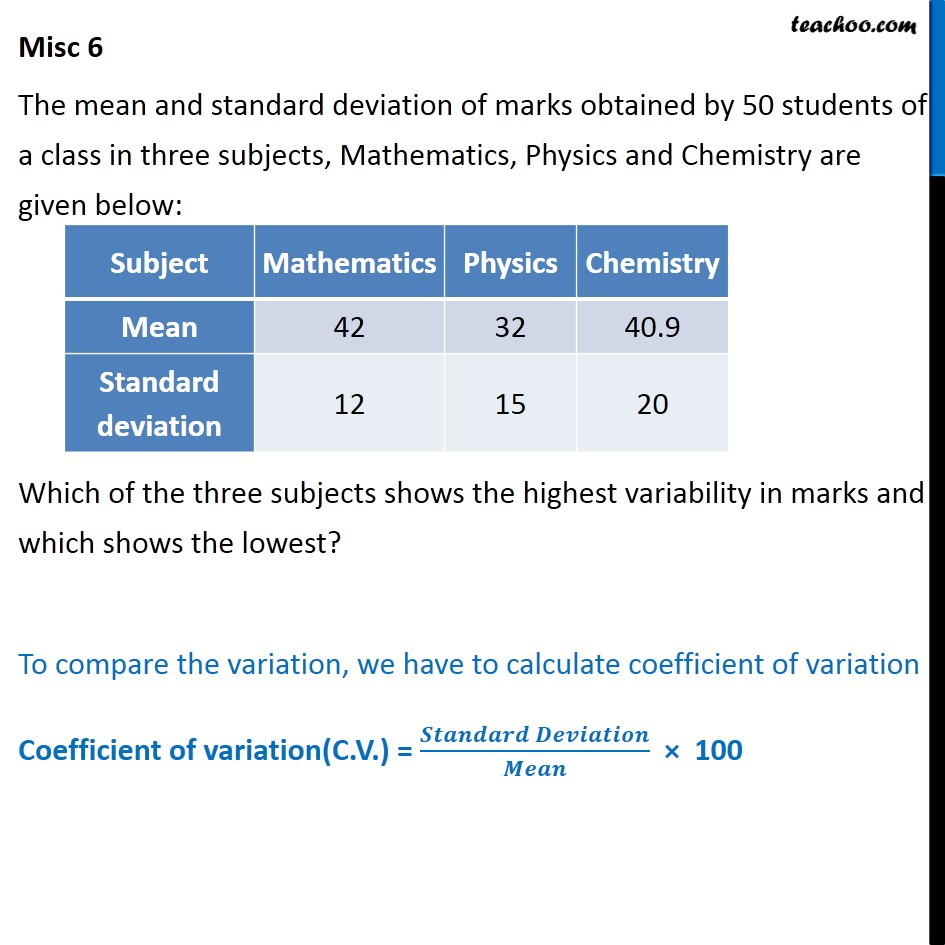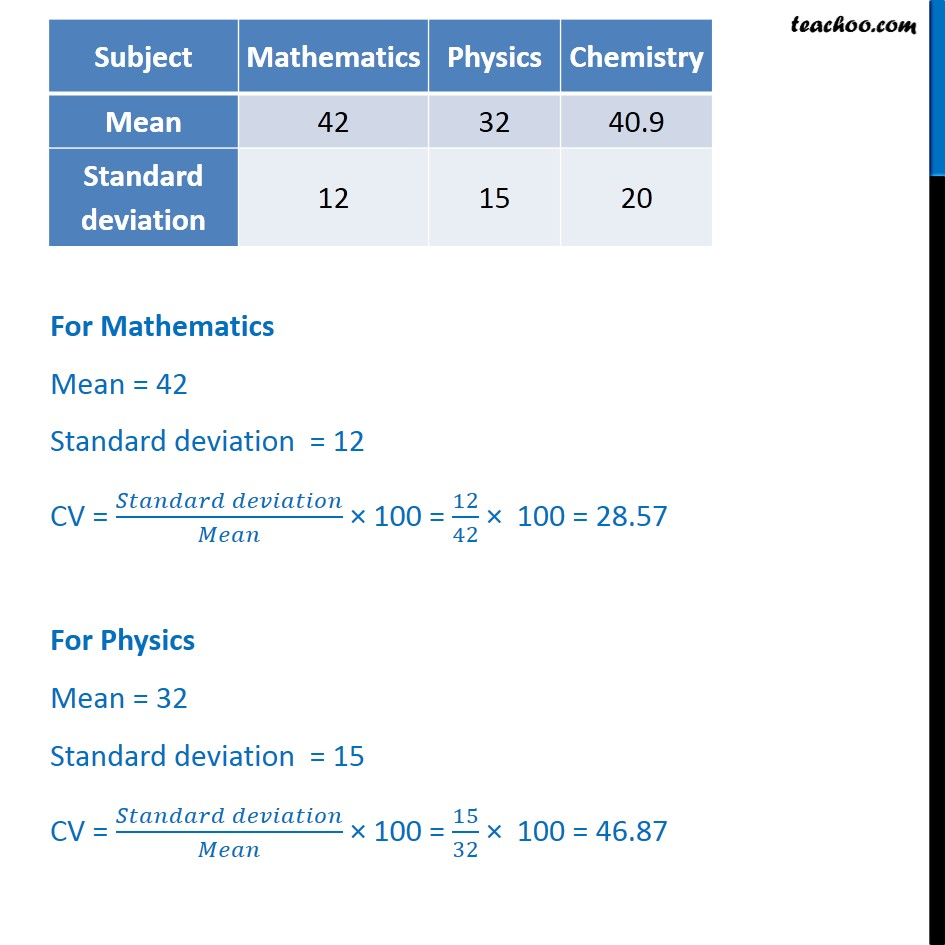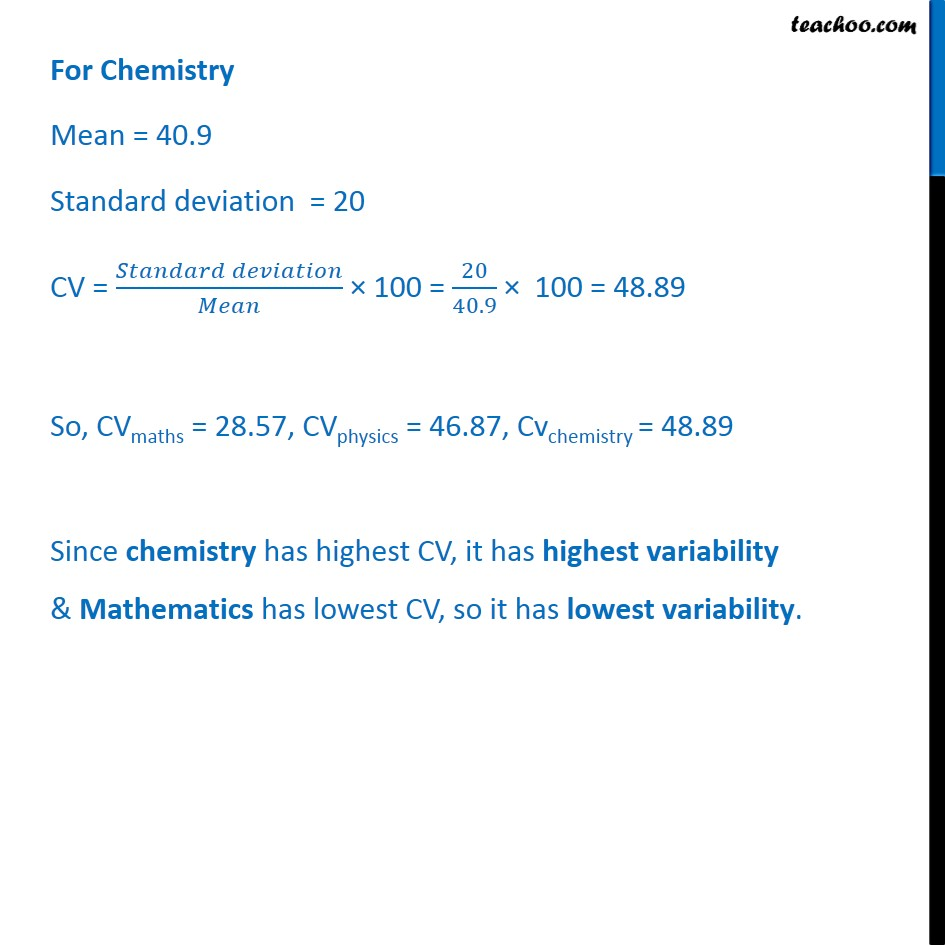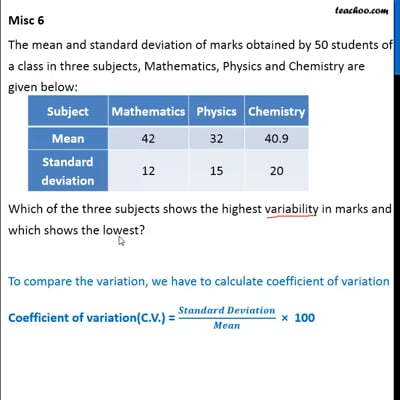Miscellaneous

Chapter 15 Class 11 Statistics (Term 1)
Serial order wiseThis video is only available for Teachoo black users

### Transcript

Misc 6 The mean and standard deviation of marks obtained by 50 students of a class in three subjects, Mathematics, Physics and Chemistry are given below: Which of the three subjects shows the highest variability in marks and which shows the lowest? To compare the variation, we have to calculate coefficient of variation Coefficient of variation(C.V.) = 𝑺𝒕𝒂𝒏𝒅𝒂𝒓𝒅 𝑫𝒆𝒗𝒊𝒂𝒕𝒊𝒐𝒏﷮𝑴𝒆𝒂𝒏﷯ × 100 For Chemistry Mean = 40.9 Standard deviation = 20 CV = 𝑆𝑡𝑎𝑛𝑑𝑎𝑟𝑑 𝑑𝑒𝑣𝑖𝑎𝑡𝑖𝑜𝑛﷮𝑀𝑒𝑎𝑛﷯ × 100 = 20﷮40.9﷯ × 100 = 48.89 So, CVmaths = 28.57, CVphysics = 46.87, Cvchemistry = 48.89 Since chemistry has highest CV, it has highest variability & Mathematics has lowest CV, so it has lowest variability.